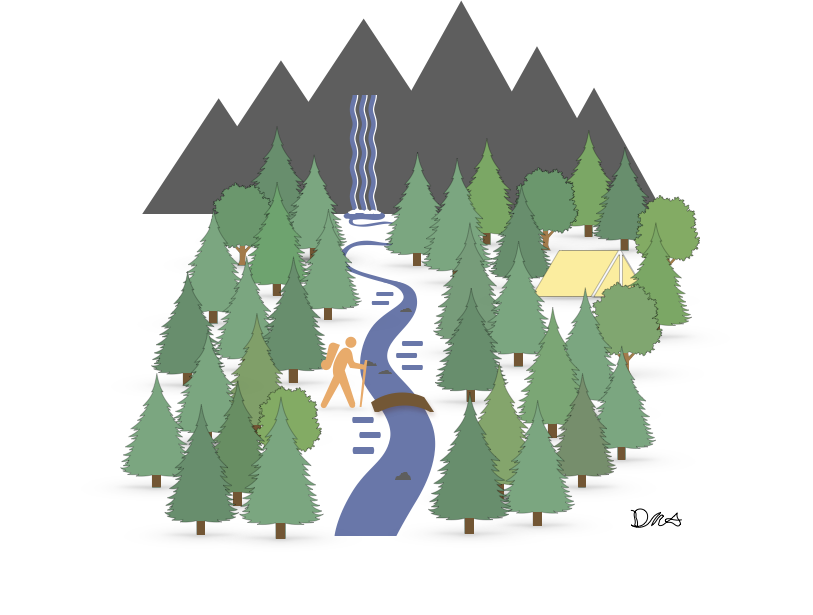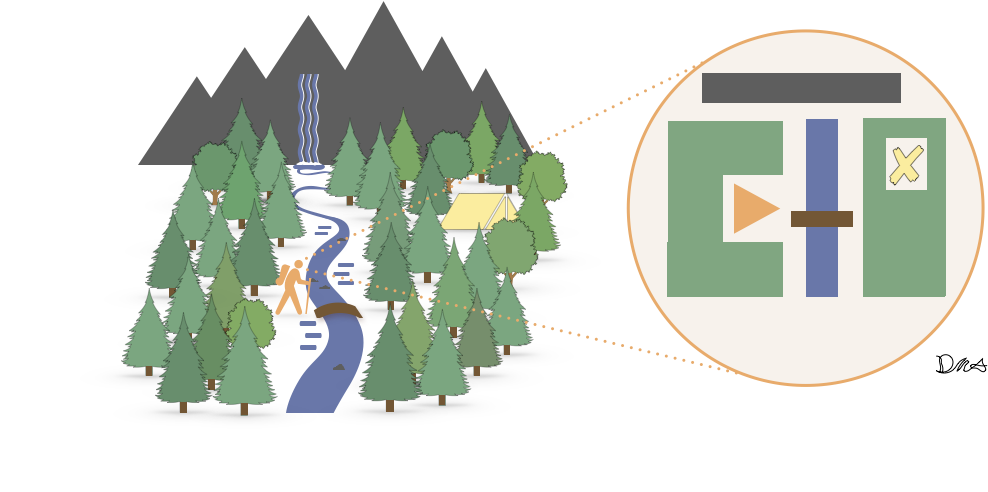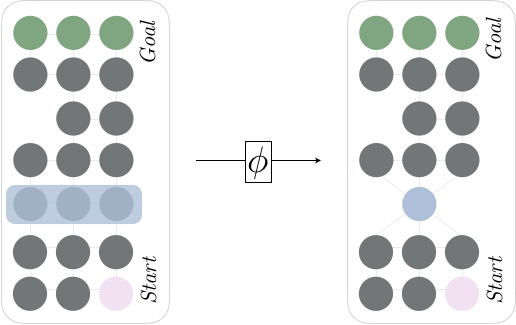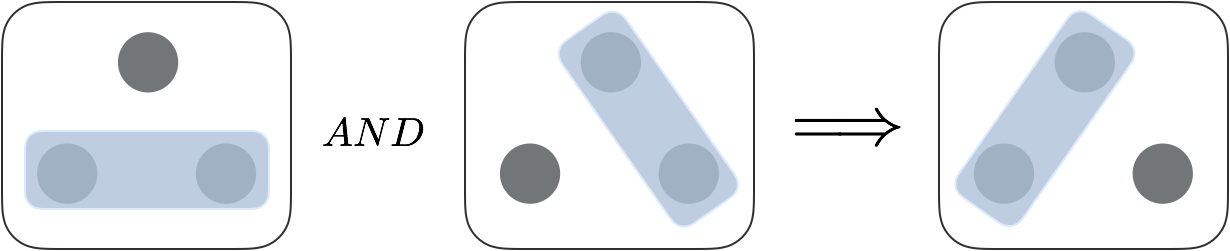# David Abel### State Abstractions for Lifelong Reinforcement Learning

##### 07/24/2018

I attended ICML in Stockholm this Summer to present two papers on Lifelong Reinforcement Learning -- in this blog post, I'll give a high level overview of our paper "State Abstractions for Lifelong Reinforcement Learning", focusing on the motivation behind the work and its central results. In this post I'll assume some familiarity with RL (things like MDPs, policies, and $$Q/V$$ functions). A big thanks to my collaborators, Dilip Arumugam, Lucas Lehnert, and my advisor Michael Littman.

For a one sentence summary: "We show how to compute state abstractions in lifelong reinforcement learning that preserve near-optimal behavior."

For more on this work, see the: Paper, Appendix, Talk, Workshop Paper.

#### 1. What Question Are We Asking?

Our goal in this work is to understand the role that abstraction plays in RL. What is abstraction? From the perspective of a decision making agent, I like to think about it in the following sense: the universe is complex and vast, but despite this complexity, its resident agents are still interested in acquiring some knowledge of their surroundings. Which knowledge? Well, whatever will be useful in making decisions that lead to more desirable possible futures of the world.

As an example, suppose you're backpacking:The above scene already contains a huge amount of information (and of course, is itself just an abstraction of a real world scenario) -- we find trees of different types, colors, and sizes, sprawled out in no easily predictable pattern. We find a waterfall, a river full of rocks and a current, a bridge, and likely plenty of wildlife, dirt, and other wonders. If we were to find ourselves in such a situation, surely we'd also enjoy the presence of things like the sun, the sky, moving clouds, the sounds of the wind, birds chirping, and the smell of the fresh air. There's a huge amount going on in just this simple scene (even ignoring the vastness of the rest of our planet, solar system, and beyond). And that's precisely the point: the world is too complex for a single agent to fully capture its workings. Still, though, we'd imagine that any agent would likely want to pick up on something about its surroundings, whether this "something" will be used to inform exploration, planning, model-free RL, policy gradient, it doesn't matter --- the point is that, by necessity, to make good decisions in this particular state of affairs, the agent must be aware of certain aspects of the world. Also by necessity, the agent can't capture everything about the world in its head. We thus come to central question of my research (and in part the focus of our paper, though of course we drill down to simpler settings and simpler tools):

My Research Agenda: How do intelligent agents come up with the right abstract understanding of the worlds they inhabit?

To return to the backpacking example, one might imagine that the agent could form a simple abstraction like the following:The depicted abstract understanding (right) captures only information about where the agent can and cannot navigate in its immediate surroundings. Further, it is potentially suggestive of abstract understanding of behavior, too, such as "crossing the bridge" a la the usual Options [Sutton, Precup, Singh 1999] story. But is this the right abstraction? Are there other, better ones? If this is the best one, why? What algorithms, inductive biases, and other considerations go into acquiring and using such abstractions?

The above defines my overarching research agenda, which naturally we're not going to completely resolve in an 8 page ICML paper. Instead, we drill into a more specific, simpler result that offers slight progress toward a solution to my broader agenda. The focus of this work is on the following question:

This Paper's Question: What is a useful state abstraction in RL, supposing an agent must solve a bunch of different tasks in the same world?

Definitions

To flesh out this question we'll need two definitions: (1) state abstraction, and (2) "solve a number of different tasks in the same world".

Definition (State Abstraction): A state abstraction is a function, $$\phi : S \rightarrow S_\phi$$, that groups the states of the original MDP into abstract states.

That is, a state abstraction imposes a clustering onto the true states of the MDP, thereby shrinking the size of the state space. Such an operation can also induce an abstract transition model and reward function, thereby yielding an abstract MDP (the MDP "in the agent's head"). As an example, consider the following application of state abstraction to a simple graph MDP:On the left, we find the "true" problem posed by the world: the agent must navigate around the graph to find the goal states in the top. If, however, the agent employs the right abstraction, we note that the environmental problem can be compressed into a slightly smaller problem, pictured on the right. This is done by choosing to ignore the horizontal coordinate for the third row; it doesn't make much of a difference to the agent to distinguish between these three states, we here choose to collapse these states into a single state. Notably this is lossy compression, as we are actually throwing away information that might be useful. However, the loss is so insignificant that we can effectively ignore it. One might ask: How lossy is the compression? For more on that, take a look at our 2016 ICML paper "Near Optimal Behavior via Approximate State Abstraction".

The final piece of the setup of our paper states that we inevestigate agents that need to solve series of tasks, not just one. The premise is that, just as we navigate a complex world (with largely fixed causal laws) and deal with a variety of different problems over our lifetime, we suppose too that our RL agents must also deal with a collection of tasks. Building on recent work in Lifelong RL, such as [Brunskill, Li 2014] and [Ruvolo, Eaton ’13], we consider the following definition of Lifelong RL:

Definition (Lifelong RL): In Lifelong RL, we fix $$S, A, \gamma$$, and repeat the following two steps indefinitely:
1. Sample a new task: $$(R_i, T_i) \sim D$$
2. Let the given agent interact with the resulting MDP, $$M_i = (S, A, R_i, T_i, \gamma)$$, for some number of steps.

Thus, over the agent's lifetime, it will be faced with many different reward and transition functions.

So, to recap, our mission is to understand how state abstractions can help with Lifelong RL.

#### 2. How did we answer the question?

The results in this paper are primarily theoretical. That is, we offer answers to our above question by introducing new mathematical structures and analysing them to show they possess desirable properties.

Our main results can be summarized as follows:

1. We introduce transitive state abstractions and show that (1) They can be efficiently computed, (2) There exist instances of transitive state abstractions that preserve near optimal behavior (so the abstract model can still capture good behavior), and (3) The most compressed transitive state abstraction has bounded compression-ratio relative to its non transitive counter part.
2. We introduce Probably Approximately Correctly State Abstractions (henceforth PAC Abstractions), inspired by PAC Learning, which defines a state abstraction that is guaranteed to be approximately correct (with high probability) with respect to a distribution over MDPs. We show PAC abstractions: (1) Can be computed from some number of sampled MDPs from the distribution, and (2) Can preserve near optimal behavior, in expectation over samples from the distribution.

To summarize: we introduce two new classes of state abstractions and show that they can be efficiently computed while still preserving near optimal behavior in lifelong RL.

Transitive Abstractions

Now, for some details. A transitive abstraction is so called because the clusterability of states must be transitive. That is, if states x and y can be clustered, and states y and z can be clustered, we can conclude that x and z can be clustered, too:The reason we care about such a property is that it can save quite a bit of computation. When we form a cluster, we are effectively looking for cliques in the graph, where an edge represents "safe clusterability". Finding the smallest clique cover in a graph is known to be NP-Hard, so we probably don't want our state abstractions to depend on this computation. Instead, we choose to invoke transitive abstractions, which can get us away from solving the full blown clique-cover problem. Instead we get clique-membership much cheaper as a result of the transitivity. This brings us to our first result:

Theorem: Transitive state abstractions can be computed efficiently

Two questions naturally follow. First: do any reasonable transitive abstractions exist? Our second result answers this question in the affirmative:

Theorem: There exist transitive state abstractions that preserve near-optimal behavior.

This flavor of result was the main focus of our 2016 ICML paper, mentioned previously. The idea is to understand whether the MDP's produced by the state abstraction are guaranteed to yield policies that will perform sufficiently well when applied in the original problem. When we saw that the abstraction "preserves near-optimal behavior", we mean that the abstracted MDP still facilitates the representation of a near-optimal policy in the original problem. In short: the abstraction doesn't throw away good behavior.

A bit more formally, we provide an upper bound on the deviation of the performance of the optimal abstract policy, denoted $$V^{\pi_\phi^*}$$, from the true optimal performance, $$V^*$$. Such results then come in the following form: $$\forall_{s \in S} : V^*(s) - V^{\pi_\phi^*}(s) \leq \tau$$ With $$\tau$$ usually a function of abstraction-specific parameters, and things like $$\gamma$$. Note that this inequality states that if we were to solve the abstracted problem to yield $$\pi_\phi^*$$ and use that policy in the original MDP, we're guaranteed to do no worse than $\tau$.

The second question asks about the degree of compression achieved by these transitive abstractions compared to their non-transitive counterparts. That is, if we were to solve the full NP-Hard problem, we're guaranteed to find the abstraction that induces the smallest possible abstracted MDP. How much larger is the transitive variant? Our third result answers this question:

Theorem: Transitive abstractions will induce an abstract state space size that is no more than $$2^{|A|}$$ times bigger than the non-transitive counterpart.
PAC Abstractions

PAC Abstractions are entirely complementary to transitive abstractions; instead, PAC abstractions are about taking an abstraction that works for a single MDP, and extending it to work for a distribution of problems. The idea is to suppose that the clustering induced by the PAC Abstraction will be correct (induce a "safe clustering") with high probability. So, if we have a PAC abstraction, we know it will (probably!) work well for a bunch of different problems.

We show two main things about PAC Abstractions:

Theorem: After some number n of sampled MDPs, we can compute a PAC abstraction, assuming access to an oracle that tells you which state-pairs are safely clusterable.
Theorem: If a given abstraction preserves near-optimal behavior in a single MDP, then its PAC extension will preserve near-optimal behavior in expectation over the distribution.

These two in some sense constitute our "main result": basically, there are ways to get near-optimal behavior out of an abstraction in the Lifelong RL setting (and we provide a recipe for computing such abstractions). The latter theorem is really about expected, value loss. That is, if an abstraction $$\phi$$ preserves near optimal behavior in a single MDP, then it will still do so in the lifelong setting (as a function of the PAC parameters $$\epsilon$$ and $$\delta$$): $$\forall_{s \in S} : E_{M \sim D}[ V_M^*(s) - V_M^{\pi_{\phi_{p,\epsilon,\delta}}^*}(s)] \leq f(\tau_p, \epsilon, \delta)$$

To compute PAC Abstractions from these n, sampled MDPs, we run roughly the following algorithm:

INPUT: $$D, \epsilon, \delta, O_p$$
OUTPUT: $$\phi_p^{\epsilon, \delta}$$

# Choose sufficiently large value of $$n$$.
0.  $$n := \ln\left(\frac{2 / \delta}{\epsilon^2}\right)$$

# Query the clustering oracle for $$n$$ samples.
1.  for $$i$$ up to $$n$$:
2.      $$M \sim D$$
3.      for each $$(s_1, s_2) \in S \times S$$:
4.          $$\hat{p}_{1,2}^i := O_p(s_1, s_2, M)$$

# Make empirical estimate about clustering probability.
5.  for each $$(s_1, s_2) \in S \times S$$:
6.      $$\hat{p}^{\epsilon, \delta}(s_1,s_2) := \text{1}\{\frac{1}{n} \sum_i \hat{p}_{1,2}^i \geq 1 - \delta\}$$

# Form clustering based on empirical estimate.
7.  for each $$(s_1, s_2) \in S \times S$$:
8.      if $$\hat{p}^{\epsilon, \delta}(s_1,s_2)$$:
9.          $$\phi_p^{\epsilon, \delta}(s_1) = \phi_p^{\epsilon, \delta}(s_2)$$

# Return.
10. return $$\phi_p^{\epsilon, \delta}$$

Most of the proof for the Theorem is about line 0 -- we use the Hoeffding Inequality to determine how large $$n$$ needs to be to ensure that we get close enough to a safe clustering. Line 9 is obscuring some detail too: the point is that we just need to place the state pairs in a cluster so that they share a cluster with all states for which the empirical estimate, $$\hat{p}(s_1,s_2)$$ is true. Lastly, the 1$$\{\ldots\}$$ notation in line 6 is denoting an indicator function.

#### A Limitation

Our final result is a surprising negative result:

Theorem: State abstractions can actually destroy learning guarantee of some learners (our result is specific to the PAC-MDP guarantee of R-Max [Brafman, Tenenholtz 2002]).

The problem comes results from how the abstract MDP is defined. As an example, consider the graph MDP I showed above. In this case, the blue circle on the right could actually induce several different variations of an abstract R and T. This is precisely the problem; if we want to preserve guarantees, these two abstract functions must be defined with respect to a fixed policy, but rarely (if ever) do agents use a fixed policy. We have some thoughts on how to fix it, but they'll be punted to another paper.

Experiments

We conduct some simple experiments to corroborate the above claims, but the real meat of the paper are the above theorems. The domains are quite simple, so no substantive conclusions should really be drawn from the empirical evidence apart from "sure, in some cases, transitive PAC abstractions can help planning and learning" (where help means reduce sample/computational complexity while preserving near-optimality).

#### 3. Conclusions, Limitations, and Next Steps

So, in summary, our big picture goal is to understand how agents can use abstraction to pick up on the right properties of the worlds they inhabit. We take "right" to mean useful in sequential decision making, so: can aid in (1) exploration, (2) transfer, (3) communication, (4) credit assignment, (5) planning, and beyond. We focus our efforts on state aggregation functions, which group the true states of the world together to form abstract states. We introduce new kinds of state abstractions that give the theoretical understanding of how to compute and use value preserving state abstractions in reinforcement learning when the agent has to solve a number of different tasks drawn from the same distribution.

I see several limitations with our work:

1. State aggregation is too simple: naturally, abstraction is a much richer process than the process of aggregation. (However, aggregation can offer insights in how to study abstraction more generally).
2. We suppose the distribution over $$(R, T)$$ is fixed -- one could imagine a more liberal definition of lifelong RL in which this assumption is not made.
3. Our experiments are quite simple: we focus on simple grid world task distributions, which offer opportunity to visualize and diagnose various abstractions, but they fall short of compelling empirical progress.
4. Our main sample bound for computing state abstractions in the lifelong setting assumes access to an unrealistic oracle; in the future we'd like to relax this assumption.

My main focus going forward is on an information theoretic view of abstraction in RL; that paper is in submission at the moment, hopefully I'll be able to share it soon.

And that's all! Thanks for reading.

-Dave